# Triangular waves

A triangular wave or triangle wave is a non-sinusoidal waveform named for its triangular shape. It is a periodic, piecewise linear, continuous real function. Like a square wave, the triangle wave contains only odd harmonics. However, the higher harmonics roll off much faster than in a square wave (proportional to the inverse square of the harmonic number as opposed to just the inverse) (Wikipedia).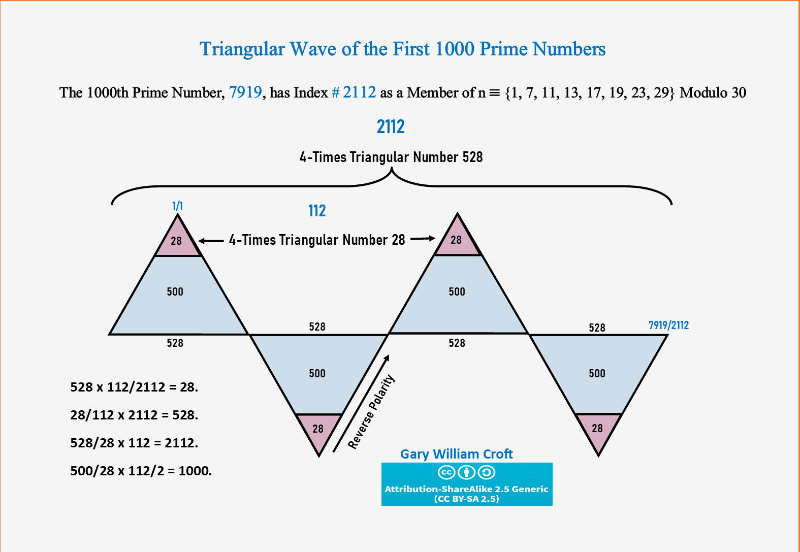Hamilton's program for proving the Poincaré conjecture involves first putting a Riemannian metric on the unknown simply connected closed 3-manifold. Such mechanism is discussed in detail by 16th prime identity.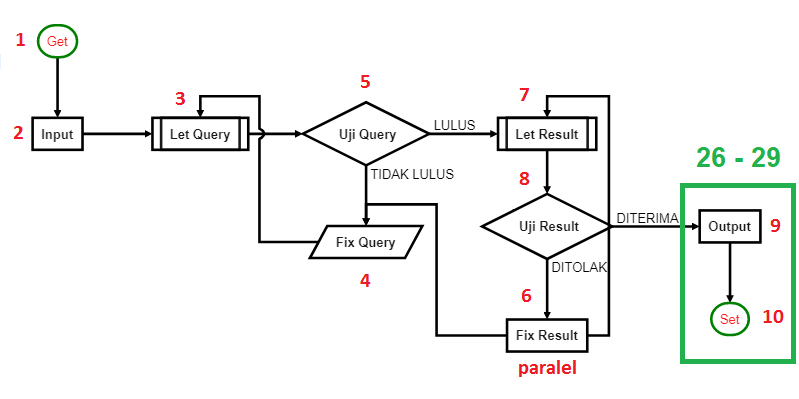There are 243 prime pairs among the first 1000 primes that sum to 7920, where 243 x 7920 gives additive sum 1,924,560 (and we note that 7920 = 22 x 360 = 2(1092 − 892), which equates to 1,924,560/(2(1092 − 892) = 243.).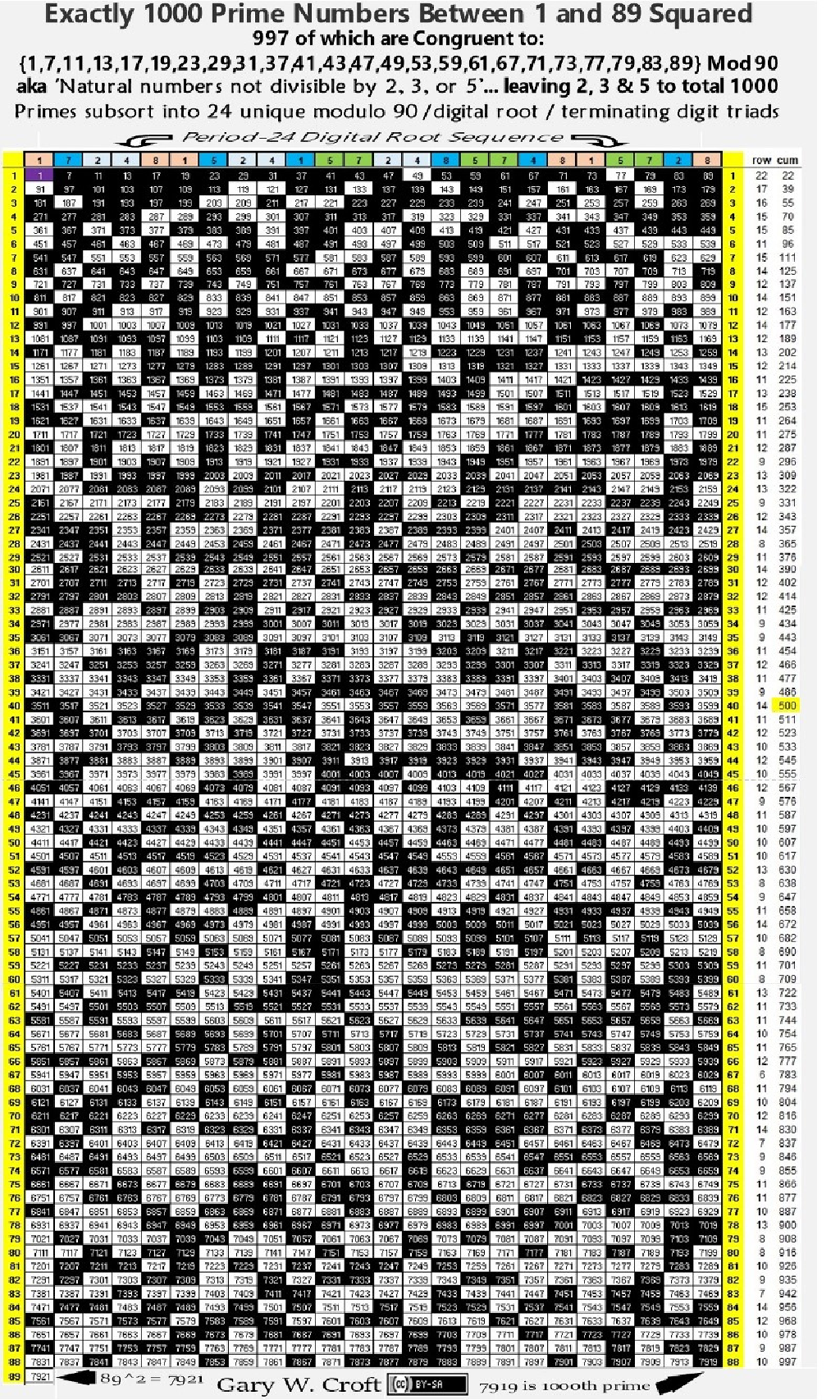Consider these two (2) numbers are laid side by side as part of Fibonacci sequence on the Pascal Triangle. Thus the above is actually represesenting the pattern of true-prime-pairs via bilateral 9 sums.

63 = 21 x 3 = 7 x 3 x 3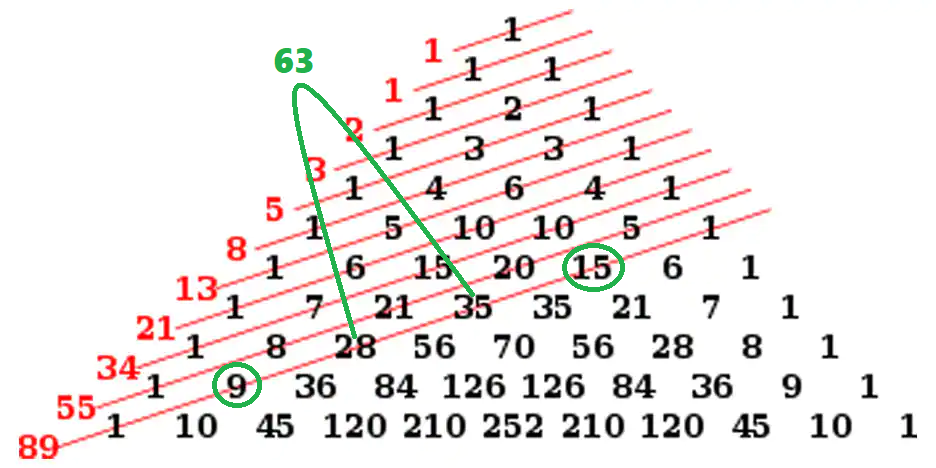Meanwhile the sum of 28 and 35, which is 63, has a digital root of 6+3 = 9 and is landed precisely on the 15's cell of the prime hexagon. So the interaction with the seven (7) objects is made through the object of 36 which has the same digital root of 9.

la position du début de l'emplacement, dans les décimales du nombre e, de la séquence 0719425863, qui est la première occurrence d'une séquence de longueur 10 contenant chaque chiffre une et une seule fois (Wikipedia).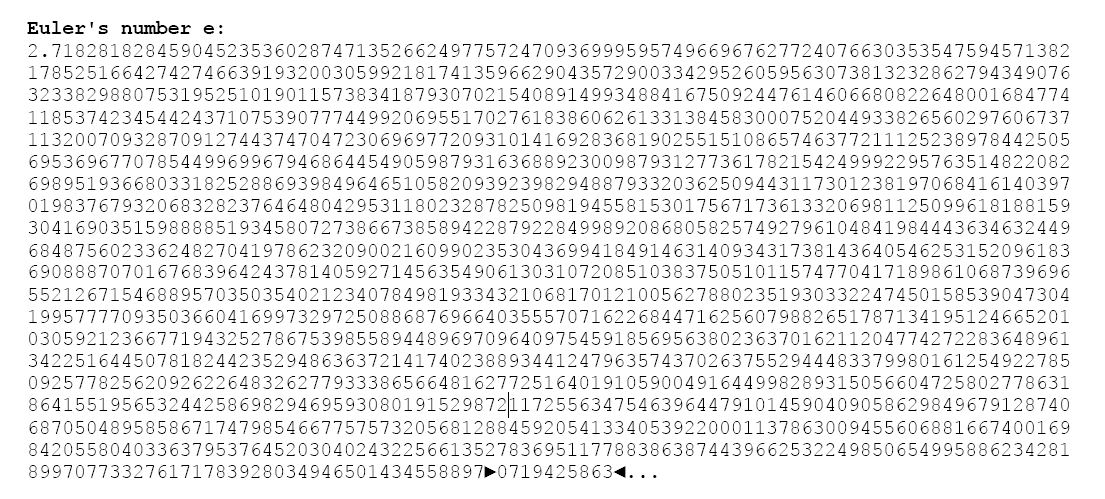This 1729 objects is passing the first cycle of True Prime Pairs so it will be located by the starting point of 286 objects of 2nd identity. This balancing will exchange 786 and 500 objects in pair.

1729 = 786 + 157 + 786 = 500 + 286 + 157 + 286 + 500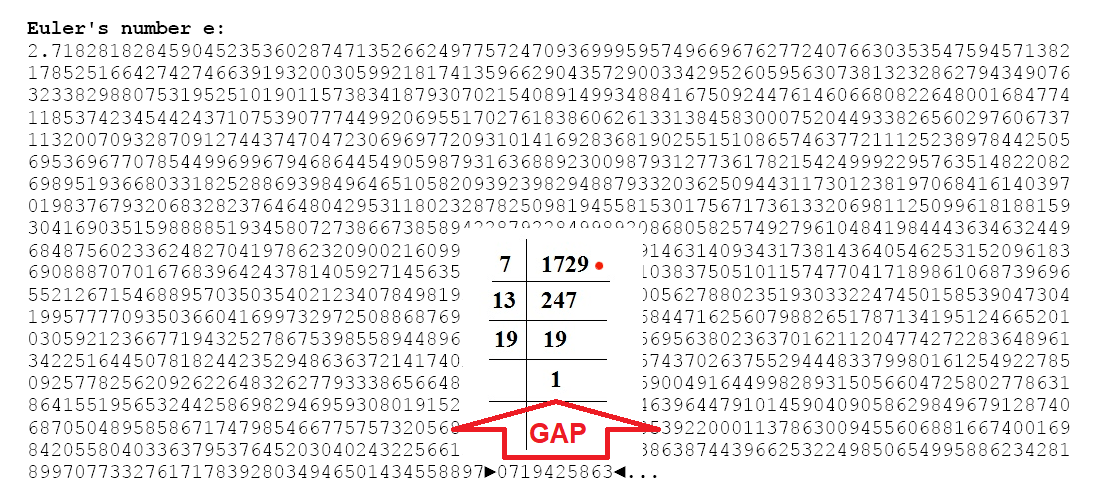The basic idea is to try to "improve" this metric; for example, if the metric can be improved enough so that it has constant positive curvature, then according to classical results in Riemannian geometry, it must be the 3-sphere.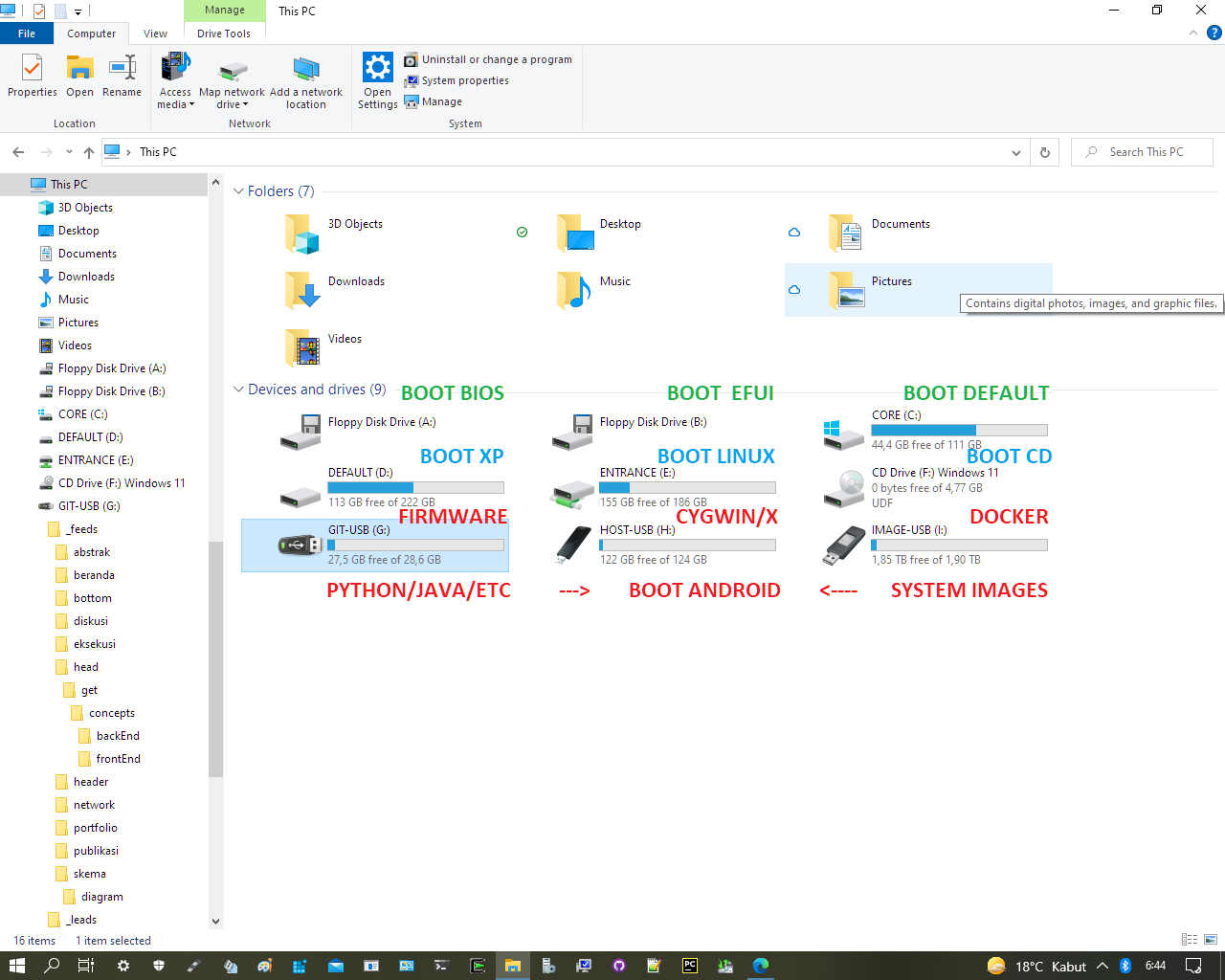Hamilton prescribed the "Ricci flow equations" for improving the metric. Ricci flow expands the negative curvature part of the manifold and contracts the positive curvature part. In some cases, Hamilton was able to show that this works

## System Images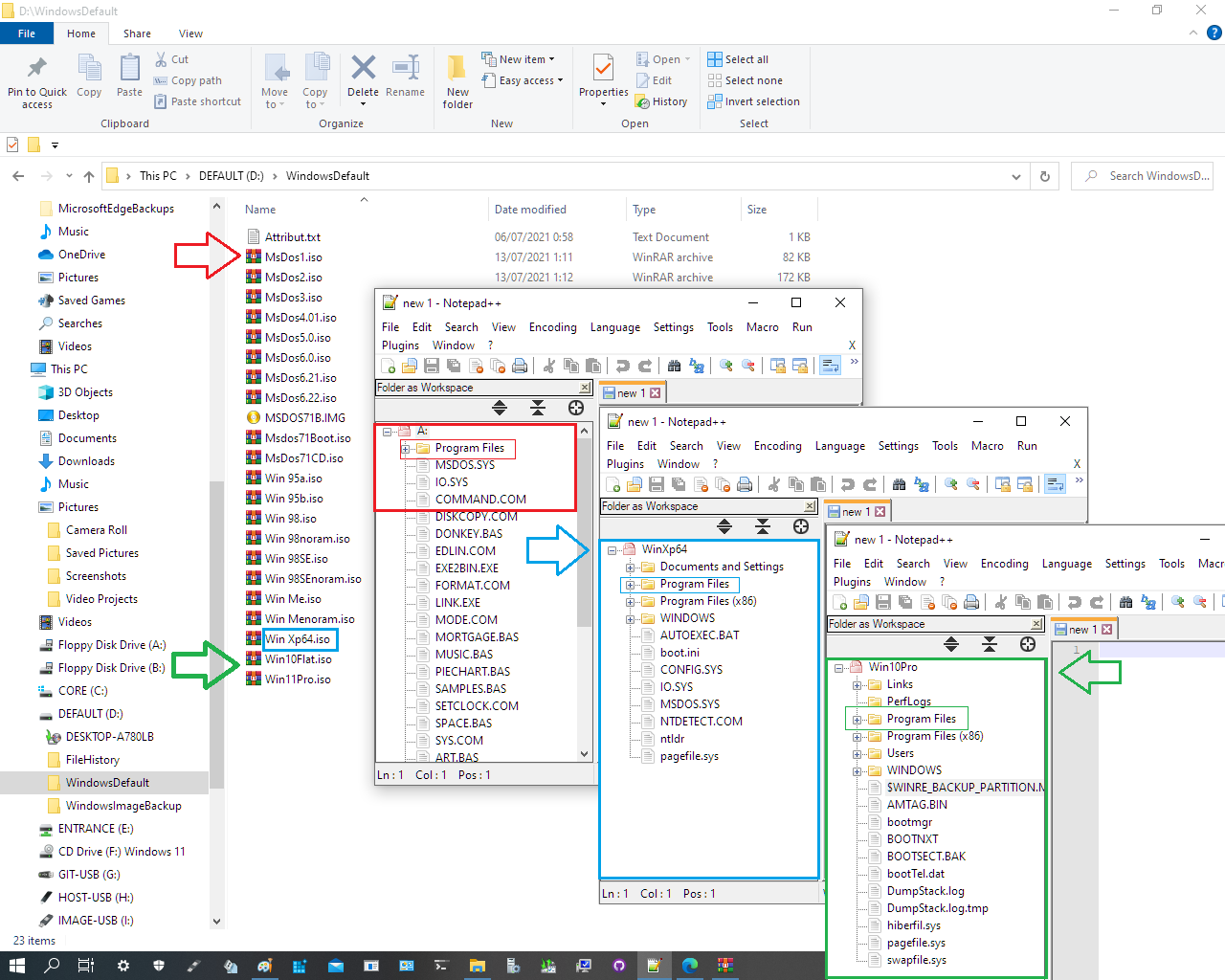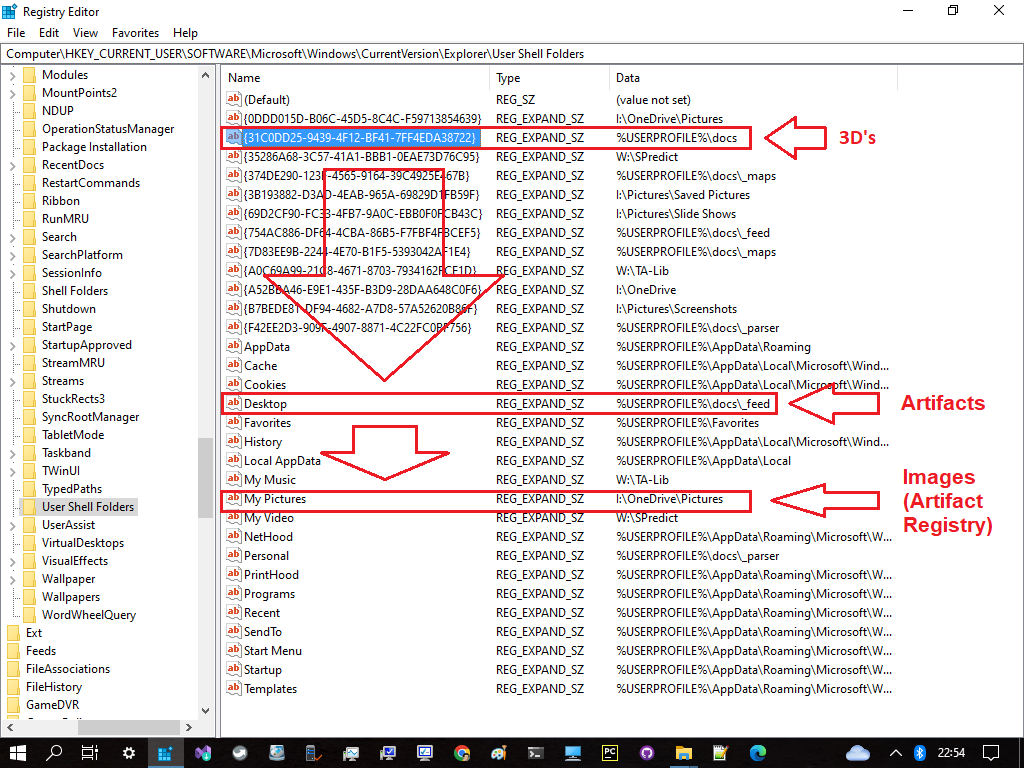Pass a startup script from Cloud Storage for the scripts greater than 256 KB.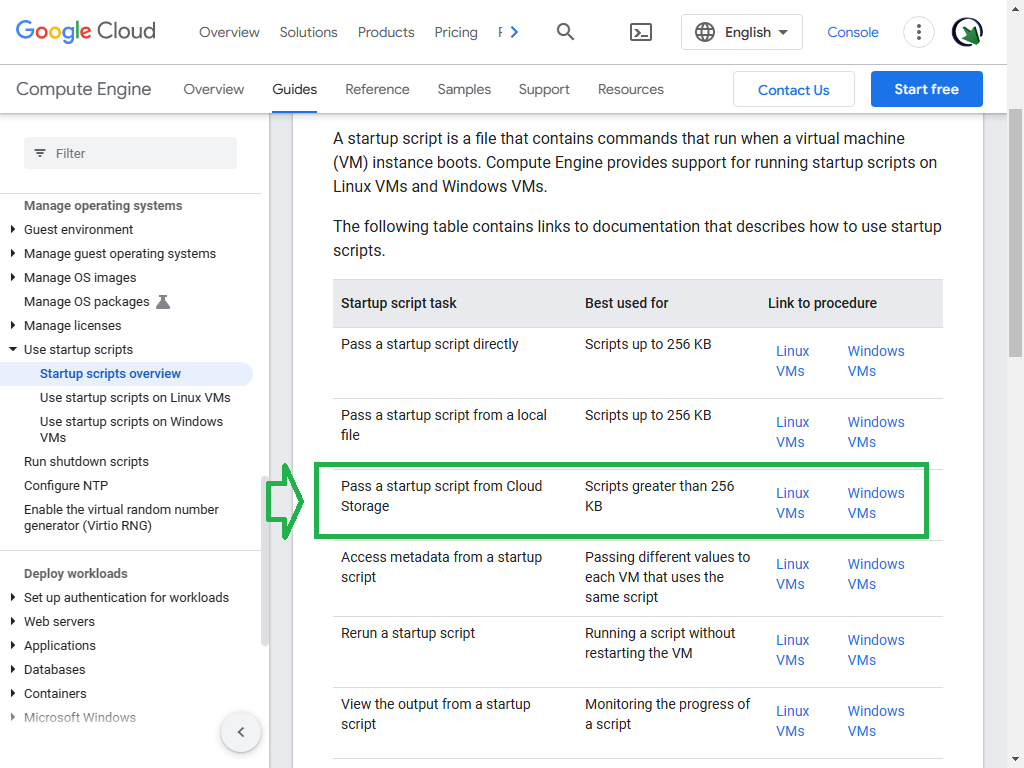Artifact Registry expands on the capabilities of Container Registry and is the recommended container registry for Google Cloud.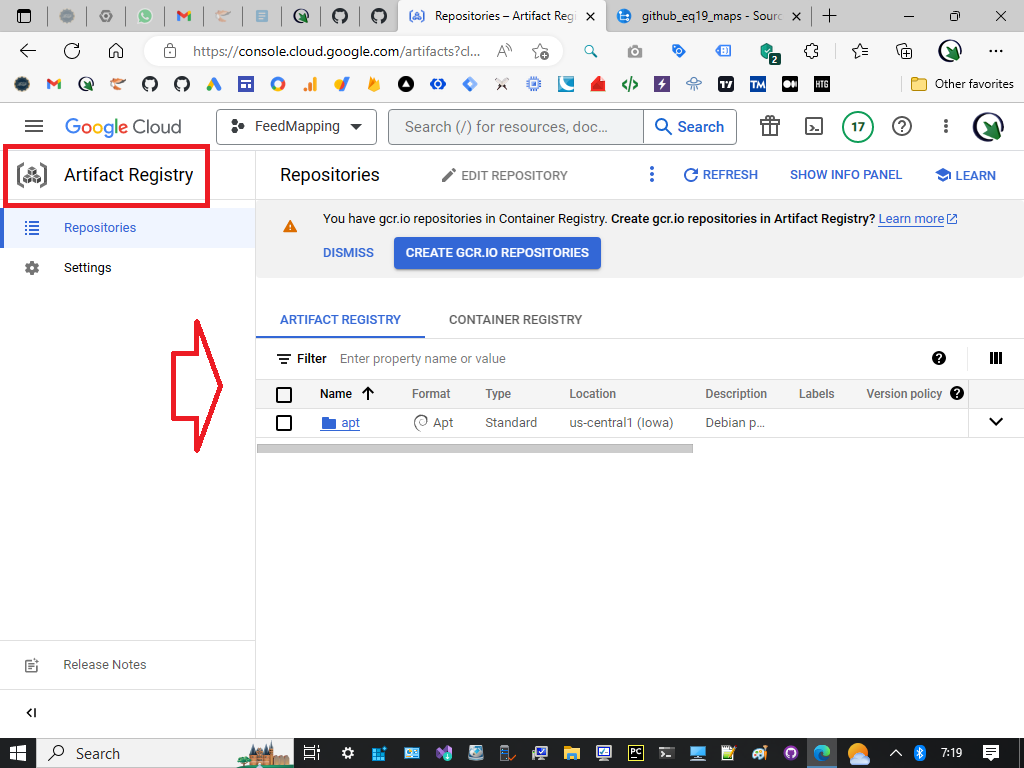A Cloud Build trigger automatically starts a build whenever you make any changes to your source code. You can configure the trigger to build your code on any changes to the source repository or only changes that match certain criteria.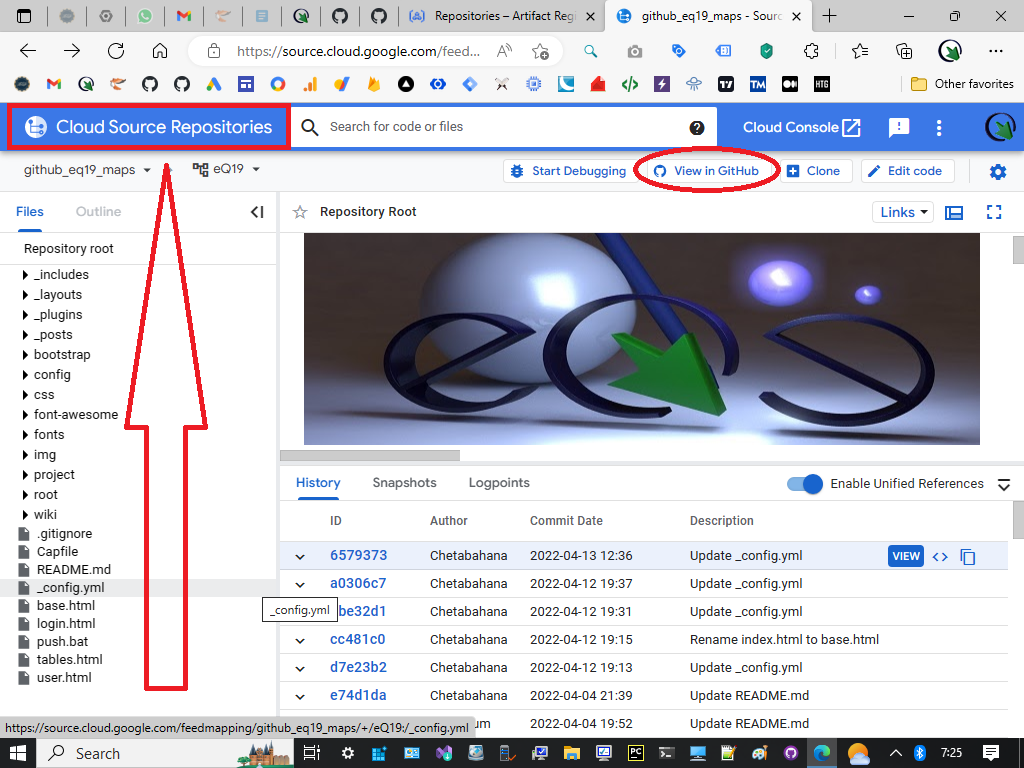Compute Engine supplies an up-to-date Container-Optimized OS (COS) image with Docker installed and launches your container when your VM starts. See below the access control for information about private registry permissions.

DNA is read by DNA polymerase in the 3′ to 5′ direction, meaning the new strand is synthesized in the 5' to 3' direction. Since the leading and lagging strand templates are oriented in opposite directions at the replication fork, **a major issue** is how to achieve synthesis of new lagging strand DNA, whose direction of synthesis is opposite to the direction of the growing replication fork (Source: Wikipedia).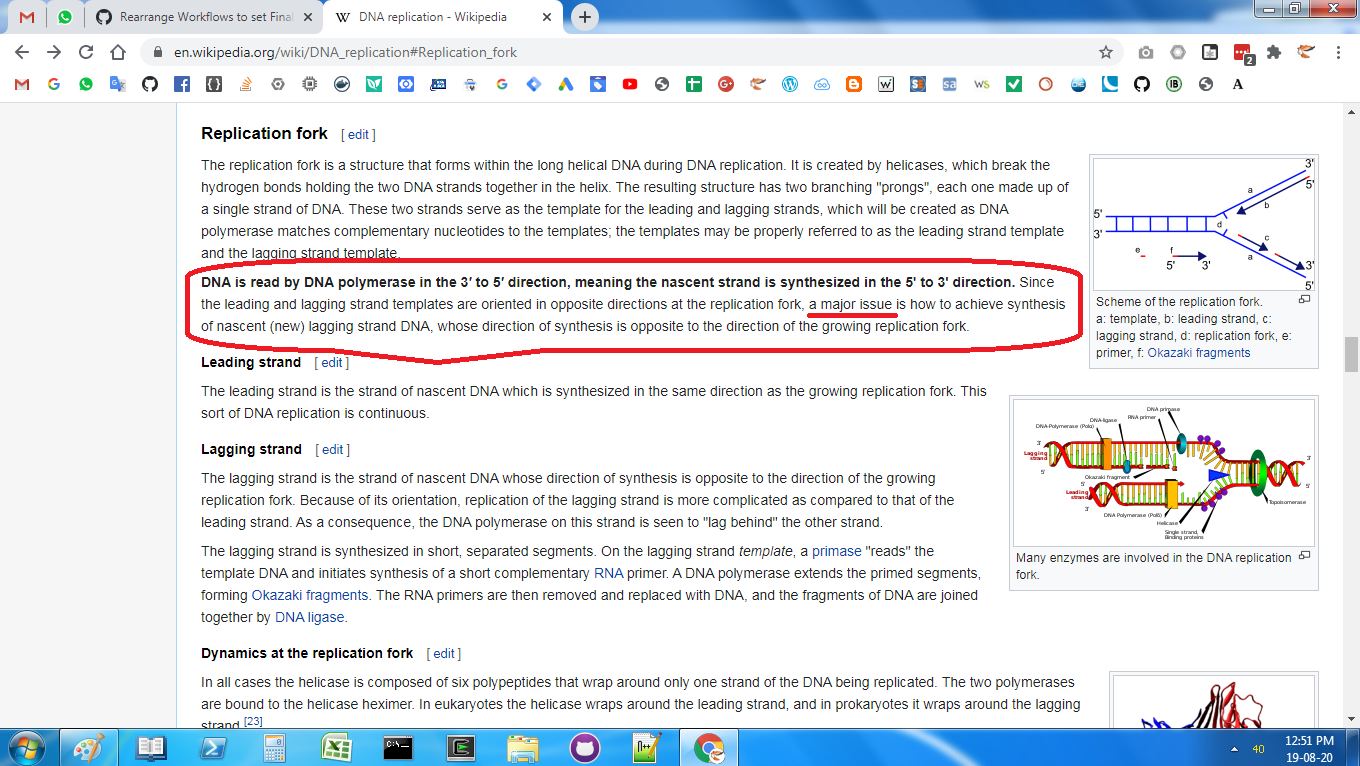Means this field remains unthoucheable. Up to the previous topic of quantum we brought you AI. A touchable intelligence. By this project we would like to introduce a brand new intelligence called UI which is the abreviation of Untouched Inteligence